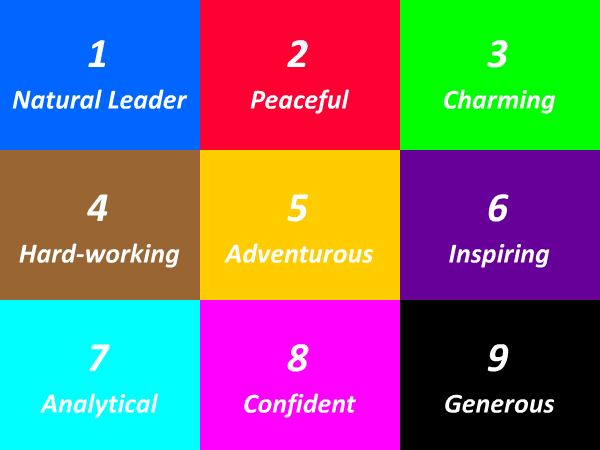# 66 Times Table - Generate Multiplication Table of 66

66 Times Table
1x66=66
2x66=132
3x66=198
4x66=264
5x66=330
6x66=396
7x66=462
8x66=528
9x66=594
10x66=660
11x66=726
12x66=792
13x66=858
14x66=924
15x66=990
16x66=1056
17x66=1122
18x66=1188
19x66=1254
20x66=1320
21x66=1386
22x66=1452
23x66=1518
24x66=1584
25x66=1650
66 Times Table
26x66=1716
27x66=1782
28x66=1848
29x66=1914
30x66=1980
31x66=2046
32x66=2112
33x66=2178
34x66=2244
35x66=2310
36x66=2376
37x66=2442
38x66=2508
39x66=2574
40x66=2640
41x66=2706
42x66=2772
43x66=2838
44x66=2904
45x66=2970
46x66=3036
47x66=3102
48x66=3168
49x66=3234
50x66=3300
66 Times Table
51x66=3366
52x66=3432
53x66=3498
54x66=3564
55x66=3630
56x66=3696
57x66=3762
58x66=3828
59x66=3894
60x66=3960
61x66=4026
62x66=4092
63x66=4158
64x66=4224
65x66=4290
66x66=4356
67x66=4422
68x66=4488
69x66=4554
70x66=4620
71x66=4686
72x66=4752
73x66=4818
74x66=4884
75x66=4950

## Test Your Skills Here

The 66 times table quiz is very useful in the improvement of kid's multiplication and maths skills. Here you will find a selection of times table tests designed to help your child to learn and practice their times tables. Fill in your answers. Once you have entered all the answers, click on 'Check' button to see whether you have got them all correct. If your answers is Wrong then we will show you the 'Correct' answer.

Practice Excersise
66 x 15 =
66 x 2 =
66 x 10 =
66 x 21 =
66 x = 924
66 x 1 =
66 x 8 =
66 x = 462
66 x = 1056
66 x = 1386#### More Tables#### Top Search Quesries for this page:

• 66 times table
• 66 times tables
• table of 66
• 66 multiplication table
• multiplication chart 66
• multiplication of 66
• 66 table maths### Spreading Knowledge Across the World

USA - United States of America  Canada  United Kingdom  Australia  New Zealand  South America  Brazil  Portugal  Netherland  South Africa  Ethiopia  Zambia  Singapore  Malaysia  India  China  UAE - Saudi Arabia  Qatar  Oman  Kuwait  Bahrain  Dubai  Israil  England  Scotland  Norway  Ireland  Denmark  France  Spain  Poland  and  many more....